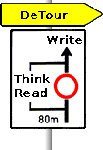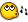# Help with dllcall to reset dllcalls getdc & createpen

## Recommended Posts

I found a script that can highlight controls. That was done by Larry and was called outlinecontrol. It's pretty cool but does not work on windows like the explorer, the autoit-helpfile and so on. Now I found the time to toy around with other scripts that do that, and I think it works just as needed. But how do I remove the old stuff? I thought resetbox() should do it, but I was wrong. What did I miss?

CODE

```; Portions written by JdeB, Valuater, Alex Peters

Opt('WinTitleMatchMode', 2)
Opt('OnExitFunc', 'Bye')

Global \$OldControl = ''
Global \$dllUser32 = DllOpen("user32.dll")
Global \$dllGdi32 = DllOpen("gdi32.dll")

While 1
\$Window = WinGetTitle('')
\$Pos1 = WinGetPos(\$Window)
\$Pos2 = WinGetClientSize(\$Window)
\$OffsetX = \$Pos1 - \$Pos2 - 3; <- BorderX +2px(to draw?) > Shell Explorer: 3/Blackbox 3
\$OffsetY = \$Pos1 - \$Pos2 - 3; <- BorderY +2px(to draw?) > Shell Explorer: 3/Blackbox 9
\$Control = ControlClassNNFromMousePos()
If \$Control <> \$OldControl Then
ResetBox()
DrawBox(\$Window, \$Control, 0x0000ff, 4, \$OffsetX, \$OffsetY)
\$OldControl=\$Control
EndIf
Sleep(100)
WEnd

Func Bye()
DllClose(\$dllUser32)
DllClose(\$dllGdi32)
EndFunc  ;==>Bye

Func ControlClassNNFromMousePos()
Local \$n = 0
Local \$ctrl_ret = ""
Local \$hWin = WinGetHandle('')
Local \$MCM = Opt("MouseCoordMode", 2)
Local \$mPos = MouseGetPos()
Opt("MouseCoordMode", \$MCM)
Local \$wPos = WinGetPos(\$hWin)
If Not IsArray(\$mPos) Or Not IsArray(\$wPos) Then Return ""
\$wPos = 0
\$wPos = 0
If Not PointInRect(\$mPos, \$wPos) Then Return ""
Local \$sClassList = @LF & WinGetClassList(\$hWin)
While \$sClassList <> @LF
\$ctrl = Pop(\$sClassList)
\$n = 1
While 1
\$wPos = ControlGetPos(\$hWin, "", \$ctrl & \$n)
If \$wPos = 0 Then ContinueLoop (2);~  just added this since some controls (like in speedcommander)
;~  won't return propper results and cause a crash right here
If PointInRect(\$mPos, \$wPos) Then \$ctrl_ret = \$ctrl & \$n
If StringInStr(\$sClassList, @LF & \$ctrl & @LF) Then
\$sClassList = StringReplace(\$sClassList, @LF & \$ctrl & @LF, @LF, 1)
\$n += 1
Else
ExitLoop
EndIf
WEnd
WEnd
Return \$ctrl_ret
EndFunc  ;==>ControlClassNNFromMousePos

Func DrawBox(\$Window, \$Control, \$LineColor, \$LineWidth, \$OffsetX = 0, \$OffsetY = 0)
\$size = WinGetPos(\$Window)
\$ControlPos = ControlGetPos(\$Window, "", \$Control)
If Not @error Then
\$ControlPos = \$ControlPos + \$size + \$OffsetX
\$ControlPos = \$ControlPos + \$size + \$OffsetY
For \$i = 1 To 4
Switch \$i
Case 1;top side
\$x1 = \$ControlPos
\$y1 = \$ControlPos
\$x2 = \$ControlPos + \$ControlPos
\$y2 = \$ControlPos
Case 2;left side
\$x1 = \$ControlPos
\$y1 = \$ControlPos
\$x2 = \$ControlPos
\$y2 = \$ControlPos + \$ControlPos
Case 3;right side
\$x1 = \$ControlPos + \$ControlPos
\$y1 = \$ControlPos
\$x2 = \$ControlPos + \$ControlPos
\$y2 = \$ControlPos + \$ControlPos
Case 4;bottom side
\$x1 = \$ControlPos
\$y1 = \$ControlPos + \$ControlPos
\$x2 = \$ControlPos + \$ControlPos
\$y2 = \$ControlPos + \$ControlPos
EndSwitch
;Below written by: Author JdeB, Co-Author Valuater
\$hd = DllCall(\$dllUser32, "int", "GetDC", "hwnd", 0)
\$pen = DllCall(\$dllGdi32, "int", "CreatePen", "int", 0, "int", \$LineWidth, "int", \$LineColor)
DllCall(\$dllGdi32, "int", "SelectObject", "int", \$hd, "int", \$pen)
DllCall(\$dllGdi32, "int", "MoveToEx", "hwnd", \$hd, "int", \$x1, "int", \$y1, "int", 0)
DllCall(\$dllGdi32, "int", "LineTo", "hwnd", \$hd, "int", \$x2, "int", \$y2)
DllCall(\$dllUser32, "int", "ReleaseDC", "hwnd", 0, "int", \$hd)
Next
EndIf
EndFunc  ;==>DrawBox

Func PointInRect(ByRef \$mPoint, ByRef \$wPoint)
If \$mPoint < \$wPoint Or _
\$mPoint < \$wPoint Or _
\$mPoint > (\$wPoint + (\$wPoint - 1)) Or _
\$mPoint > (\$wPoint + (\$wPoint - 1)) Then Return 0
Return 1
EndFunc  ;==>PointInRect

Func Pop(ByRef \$szList)
Local \$buff = StringTrimLeft(\$szList, 1)
\$buff = StringLeft(\$buff, StringInStr(\$buff, @LF) - 1)
\$szList = StringTrimLeft(\$szList, StringLen(\$buff) + 1)
Return \$buff
EndFunc  ;==>Pop

Func ResetBox()
Local \$WTMM = Opt("WinTitleMatchMode",4)
Opt("WinTitleMatchMode",\$WTMM)
\$hwnd = WinGetHandle(\$Window,'')
ConsoleWrite('@@ Debug(61) : \$hwnd = ' & \$hwnd & @lf & '>Error code: ' & @error & @lf);### Debug Console
DLLCall(\$dllUser32,"int","InvalidateRect","hwnd",\$hwnd,"int",0,"int",1)
EndFunc```

Edited by dabus

##### Share on other sites

Yes, this works: Just Replace the ResetBox Func with code borrowed from matrix200.CODE

```; Portions written by JdeB, Valuater, Alex Peters

Opt('WinTitleMatchMode', 2)
Opt('OnExitFunc', 'Bye')

Global \$OldControl = '', \$hd
Global \$dllUser32 = DllOpen("user32.dll")
Global \$dllGdi32 = DllOpen("gdi32.dll")

While 1
\$Window = WinGetTitle('')
\$Pos1 = WinGetPos(\$Window)
If @error=1 Then ContinueLoop
\$Pos2 = WinGetClientSize(\$Window)
If @error=1 Then ContinueLoop
\$OffsetX = \$Pos1 - \$Pos2 - 3; <- BorderX +2px(to draw?) > Shell Explorer: 3/Blackbox 3
\$OffsetY = \$Pos1 - \$Pos2 - 9; <- BorderY +2px(to draw?) > Shell Explorer: 3/Blackbox 9
\$Control = ControlClassNNFromMousePos()
If \$Control <> \$OldControl Then
ForceRefresh(\$Window)
DrawBox(\$Window, \$Control, 0x0000ff, 4, \$OffsetX, \$OffsetY)
\$OldControl=\$Control
EndIf
Sleep(100)
WEnd

Func Bye()
DllClose(\$dllUser32)
DllClose(\$dllGdi32)
EndFunc  ;==>Bye

Func ControlClassNNFromMousePos()
Local \$n = 0
Local \$ctrl_ret = ""
Local \$hWin = WinGetHandle('')
Local \$MCM = Opt("MouseCoordMode", 2)
Local \$mPos = MouseGetPos()
Opt("MouseCoordMode", \$MCM)
Local \$wPos = WinGetPos(\$hWin)
If Not IsArray(\$mPos) Or Not IsArray(\$wPos) Then Return ""
\$wPos = 0
\$wPos = 0
If Not PointInRect(\$mPos, \$wPos) Then Return ""
Local \$sClassList = @LF & WinGetClassList(\$hWin)
While \$sClassList <> @LF
\$ctrl = Pop(\$sClassList)
\$n = 1
While 1
\$wPos = ControlGetPos(\$hWin, "", \$ctrl & \$n)
If \$wPos = 0 Then ContinueLoop (2);~  just added this since some controls (like in speedcommander)
;~  won't return propper results and cause a crash right here
If PointInRect(\$mPos, \$wPos) Then \$ctrl_ret = \$ctrl & \$n
If StringInStr(\$sClassList, @LF & \$ctrl & @LF) Then
\$sClassList = StringReplace(\$sClassList, @LF & \$ctrl & @LF, @LF, 1)
\$n += 1
Else
ExitLoop
EndIf
WEnd
WEnd
Return \$ctrl_ret
EndFunc  ;==>ControlClassNNFromMousePos

Func DrawBox(\$Window, \$Control, \$LineColor, \$LineWidth, \$OffsetX = 0, \$OffsetY = 0)
\$size = WinGetPos(\$Window)
\$ControlPos = ControlGetPos(\$Window, "", \$Control)
If Not @error Then
\$ControlPos = \$ControlPos + \$size + \$OffsetX
\$ControlPos = \$ControlPos + \$size + \$OffsetY
For \$i = 1 To 4
Switch \$i
Case 1;top side
\$x1 = \$ControlPos
\$y1 = \$ControlPos
\$x2 = \$ControlPos + \$ControlPos
\$y2 = \$ControlPos
Case 2;left side
\$x1 = \$ControlPos
\$y1 = \$ControlPos
\$x2 = \$ControlPos
\$y2 = \$ControlPos + \$ControlPos
Case 3;right side
\$x1 = \$ControlPos + \$ControlPos
\$y1 = \$ControlPos
\$x2 = \$ControlPos + \$ControlPos
\$y2 = \$ControlPos + \$ControlPos
Case 4;bottom side
\$x1 = \$ControlPos
\$y1 = \$ControlPos + \$ControlPos
\$x2 = \$ControlPos + \$ControlPos
\$y2 = \$ControlPos + \$ControlPos
EndSwitch
;Below written by: Author JdeB, Co-Author Valuater
\$hd = DllCall(\$dllUser32, "int", "GetDC", "hwnd", 0)
\$pen = DllCall(\$dllGdi32, "int", "CreatePen", "int", 0, "int", \$LineWidth, "int", \$LineColor)
DllCall(\$dllGdi32, "int", "SelectObject", "int", \$hd, "int", \$pen)
DllCall(\$dllGdi32, "int", "MoveToEx", "hwnd", \$hd, "int", \$x1, "int", \$y1, "int", 0)
DllCall(\$dllGdi32, "int", "LineTo", "hwnd", \$hd, "int", \$x2, "int", \$y2)
DllCall(\$dllUser32, "int", "ReleaseDC", "hwnd", 0, "int", \$hd)
Next
EndIf
EndFunc  ;==>DrawBox

Func PointInRect(ByRef \$mPoint, ByRef \$wPoint)
If \$mPoint < \$wPoint Or _
\$mPoint < \$wPoint Or _
\$mPoint > (\$wPoint + (\$wPoint - 1)) Or _
\$mPoint > (\$wPoint + (\$wPoint - 1)) Then Return 0
Return 1
EndFunc  ;==>PointInRect

Func Pop(ByRef \$szList)
Local \$buff = StringTrimLeft(\$szList, 1)
\$buff = StringLeft(\$buff, StringInStr(\$buff, @LF) - 1)
\$szList = StringTrimLeft(\$szList, StringLen(\$buff) + 1)
Return \$buff
EndFunc  ;==>Pop

Func ForceRefresh(\$hWnd, \$rRect=0, \$bErase=0)
Local \$pRect, \$aResult
if \$rRect <> 0 then \$pRect = DllStructGetPtr(\$rRect)
\$aResult = DllCall("User32.dll", "int", "InvalidateRect", "hwnd", \$hWnd, "ptr", \$pRect, "int", 0)
EndFunc```

Edited by dabus

## Create an account

Register a new account

• ### Recently Browsing   0 members

×

• Wiki

• Back

• #### Beta

• Git
• FAQ
• Our Picks
×
• Create New...